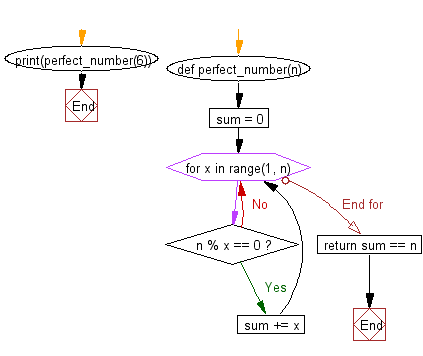﻿ Python Exercise: Check whether a number is perfect or not - w3resource# Python Exercise: Check whether a number is perfect or not

## Python Functions: Exercise-11 with Solution

Write a Python function to check whether a number is perfect or not.

According to Wikipedia : In number theory, a perfect number is a positive integer that is equal to the sum of its proper positive divisors, that is, the sum of its positive divisors excluding the number itself (also known as its aliquot sum). Equivalently, a perfect number is a number that is half the sum of all of its positive divisors (including itself).
Example : The first perfect number is 6, because 1, 2, and 3 are its proper positive divisors, and 1 + 2 + 3 = 6. Equivalently, the number 6 is equal to half the sum of all its positive divisors: ( 1 + 2 + 3 + 6 ) / 2 = 6. The next perfect number is 28 = 1 + 2 + 4 + 7 + 14. This is followed by the perfect numbers 496 and 8128.

Sample Solution:-

Python Code:

``````def perfect_number(n):
sum = 0
for x in range(1, n):
if n % x == 0:
sum += x
return sum == n
print(perfect_number(6))
```
```

Sample Output:

```True
```

Pictorial presentation:Flowchart:## Visualize Python code execution:

The following tool visualize what the computer is doing step-by-step as it executes the said program:

Python Code Editor:

Have another way to solve this solution? Contribute your code (and comments) through Disqus.

What is the difficulty level of this exercise?

Test your Python skills with w3resource's quiz

﻿

## Python: Tips of the Day

Python: Yield Statement

You can think of yield statement in the same category as the return statement. The difference is, while return statement returns a value and the function ends, yield statement can return a sequence of values, it sort of yields, hence the name.

If you're interested in algorithms, here is a nice demonstration of Bubble Sort Algorithm Visualization where you can see how yield is needed and used.

```def f1(x):
yield x
yield x**3
yield x**4
print (f1(5))
for j in f1(5):
print (j)
```

Output:

```5
125
625```
```def f2(b):
for j in range(b):
yield j
for j in f2(4):
print(j)
```

Output:

```0
1
2
3```